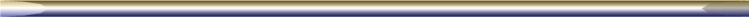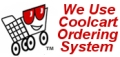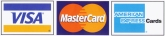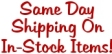Our customers find it useful in ordering mercury contactors and relays if they can interpret part numbers from various manufacturers. In the near future we  will be expanding this page to accommodate as many kinds of part numbers as possible. Below we have outlined what has become an unofficial standard for these numbers.    (Also See "Tilt Switch" part number page)Example : 235NO-120AH First number : "2" = The amount of poles (max of three). Second two or three numbers : "35"  = Amperage Rating. Two Letters denote output /contact type :                 N.O. = Normally Open      N.C. = Normally Closed The last part of the part number denotes the coil voltage :                 120AH = 120 Volts AC    24VDC = 24 Volts DC Note: The above example assumes at least a two pole relay. If the relay in question is a single pole, then the first number would drop off. Leaving 35NO-120AH denoting a single pole relay. Note: If the part number begins with 4 numbers, then this normally denotes a relay in the family of 100 Amp or above. Example would be 3100NO-120AH. This would be a 3 pole, 100 Amp relay contactor. Again 100NO-120AH would be a single pole, 100 Amp relay.  Page links for buying online are below.
 Site Map is here.